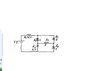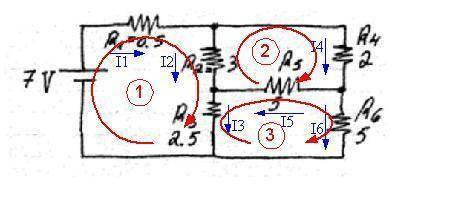# Find the Electric current

• omni

#### omni

given a electrical network (in the picture)
i asked to find all the current in each resistor.
so for the start i found the RT,i got RT=3.5-ohm (i did it with the Y-delta transform).
then i calculate IT and i got IT=2A.
all i know is R1=2A.
but my problem is how to find the others current.

thanks

#### Attachments

•dfdfd.JPG
12.9 KB · Views: 399
Why don't you write loop equations and solve for the loop currents?

well, i really tried to do it with the loop equations but i not get it.
i know the Kirchhoff's current law and the Kirchhoff's voltage law.
i also know that I1=I4+I2.
but how to work out the loop equations this i not get it yet.

thanks.

omni said:
well, i really tried to do it with the loop equations but i not get it.
i know the Kirchhoff's current law and the Kirchhoff's voltage law.
i also know that I1=I4+I2.
but how to work out the loop equations this i not get it yet.

thanks.

Why don't you show what you wrote for the loop equations so that we can see what happened?

I1=I4+I2.

+7-0.5*I1-3*I2-2.5*I3=0v

+7-3*I2-2*I4-5*I5=0V

+7-5*I5-5*I6-2.5*I3=0v

omni said:
I1=I4+I2.

+7-0.5*I1-3*I2-2.5*I3=0v

+7-3*I2-2*I4-5*I5=0V

+7-5*I5-5*I6-2.5*I3=0v

You've got loop problemsFrom what I can tell from your equations the 7V source should only appear in ONE of the loops you've used (If the 7V supply is in a loop, then R1 which is in series with it must also appear in that loop); it should not appear in all the loops if the loop path does not pass through it. It is possible to choose three loops that would each include the 7V source, but you didn't do that.

Here's your circuit diagram again but with what I imagine you've set as the currents and loops.It is important to remember that after assigning current directions that you respect those directions when traversing your loop and adding up the voltage drops or rises. If the path passes through a resistor in the same direction as its current, then there is a voltage drop. If the current is opposite to the path direction then there is a voltage rise through that resistor.

#### Attachments

hey thanks for the help.
"You've got loop problems From what I can tell from your equations the 7V source should only appear in ONE of the loops " GERAT INFO.

is possible that you can show me the way to find for example I2 or any In. ?

and then i hope i will understand more good the way to the answer.

thanks.

omni said:
hey thanks for the help.
"You've got loop problems From what I can tell from your equations the 7V source should only appear in ONE of the loops " GERAT INFO.

is possible that you can show me the way to find for example I2 or any In. ?

and then i hope i will understand more good the way to the answer.

thanks.

I can't provide a direct solution for you (Forum rules), but I can get you started by showing you how to write one of the loop equations.

Let's take the loop I've labelled as (2) in the diagram. Starting at the node where R2 and R5 meet and walking clockwise around the loop I see:

1. R2 with its labelled current I2 flowing AGAINST my progress, so that's a voltage rise: I2*3
2. R4 with its current flowing in the same direction that I'm going, so that's a voltage drop: I4*2
3. R5 with its current flowing in the same direction I'm going, so that's a voltage drop: I5*5

Putting them together gives: I2*3 - I4*2 - I5*5 = 0

Do the same thing for all three loops. Next you'll have to write the KCL node equations so that you have the relationships between the currents (sum of currents flowing into a give node = sum of currents flowing out).

ok i did the 2 others loop equations, and this what i got:

for what you labelled as (1) i got:7v-(I1*0.5+I2*3+I3*2.5)=0
for what you labelled as (3) i got: I6*5-I5*5-I3*2.5=0

did i correct till here ?

omni said:
ok i did the 2 others loop equations, and this what i got:

for what you labelled as (1) i got:7v-(I1*0.5+I2*3+I3*2.5)=0
for what you labelled as (3) i got: I6*5-I5*5-I3*2.5=0

did i correct till here ?

Yes, they look good.

Now you'll need a few KCL equations at nodes in order to describe the particular branching relationships between the currents.

hi.
thank you very much.
i solve the problem :)

ok thank you. :)

omni said:
ok thank you. :)

You're very welcome